# High School Chemistry : Calculating pH and pOH

## Example Questions

2 Next →

### Example Question #1 : P H

Acids and bases can be described in three principal ways. The Arrhenius definition is the most restrictive. It limits acids and bases to species that donate protons and hydroxide ions in solution, respectively. Examples of such acids include HCl and HBr, while KOH and NaOH are examples of bases. When in aqueous solution, these acids proceed to an equilibrium state through a dissociation reaction.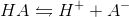All of the bases proceed in a similar fashion.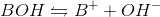The Brønsted-Lowry definition of an acid is a more inclusive approach. All Arrhenius acids and bases are also Brønsted-Lowry acids and bases, but the converse is not true. Brønsted-Lowry acids still reach equilibrium through the same dissociation reaction as Arrhenius acids, but the acid character is defined by different parameters. The Brønsted-Lowry definition considers bases to be hydroxide donors, like the Arrhenius definition, but also includes conjugate bases such as the A- in the above reaction. In the reverse reaction, A- accepts the proton to regenerate HA. The Brønsted-Lowry definition thus defines bases as proton acceptors, and acids as proton donors.

A scientist is studying an aqueous sample of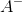, and finds that the hydroxide concentration is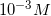. Which of the following is true?

The pH of the solution is 11

The pH of the solution cannot be determined

The pH of the solution is 3

The pOH of the solution is 11

The concentration of protons is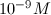The pH of the solution is 11

Explanation:

Given the hydroxide ion concentration, we will need to work using pOH to find the pH. We know that the sum of pH and pOH is equal to 14.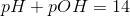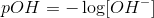Use our value for the concentration to find the pOH.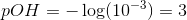Now that we have the pOH, we can use it to solve for the pH.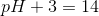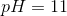### Example Question #11 : Calculating P H And P Oh

An arterial blood sample from a patient has a pH of 7.4. One day later, the same patient has an arterial blood pH of 7.15. How many times more acidic is the patient's blood on the second day?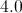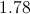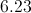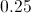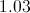Explanation:

The equation to calculate pH is: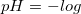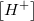The normal pH of arterial blood is around 7.4. This reflects a concentration of hydrogen ions that can be found using the pH equation.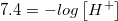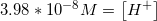Using similar calculations for the second blood sample, we can find the hydrogen ion concentration again.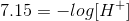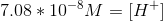Now that we have both concentrations, can find the ratio of the acidity of the two samples.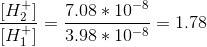You may know from biological sciences that this is approaching a lethal level of acidosis.

### Example Question #22 : Acids And Bases

You find a bottle in a lab that has a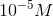solution of acid. The acid has the following dissociative properties: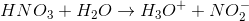What is the pH of this solution?Explanation: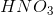is a strong acid, meaning it will completely dissociate in solution. As such, the concentration of the acid will be equal to the proton concentration. Thus, to find pH, you should just plug the molar concentration of the acid solution into the pH formula.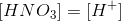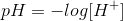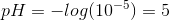### Example Question #11 : Calculating P H And P Oh

Calculate the pOH and hydroxide ion concentration in an organism whose blood pH was measured to be 8.00 at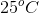.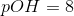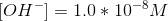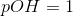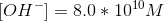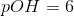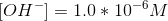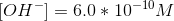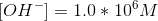Explanation:

To find the pOH note that. Therefore one can solve for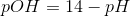. Plug in the value of the organism's blood pH and solve to getWe find the concentration of hydroxide ions based on the formula for pOH: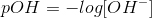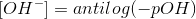.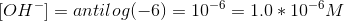### Example Question #12 : Calculating P H And P Oh

Calculate the pH of the following solution at: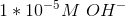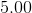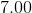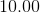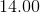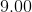Explanation:

Use the the dissociation constant for waterto calculate the concentration of hydrogen ions and then convert to the pH scale.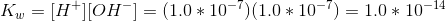Rearrange the dissociation constant to solve for the hydrogen ion concentration: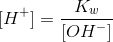Plug in the given concentrations to find the actual hydrogen ion concentration: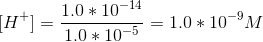Convert the hydrogen ion concentration to pH scale: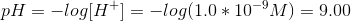### Example Question #13 : Calculating P H And P Oh

Calculate the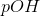of a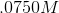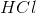solution.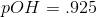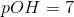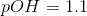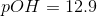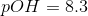Explanation:

Relevant equations: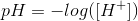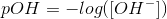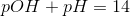is a strong acid, so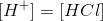Combine equations: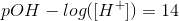Plug in values: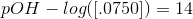2 Next →

### All High School Chemistry Resources